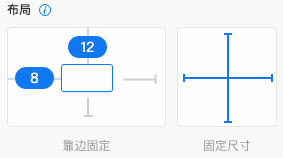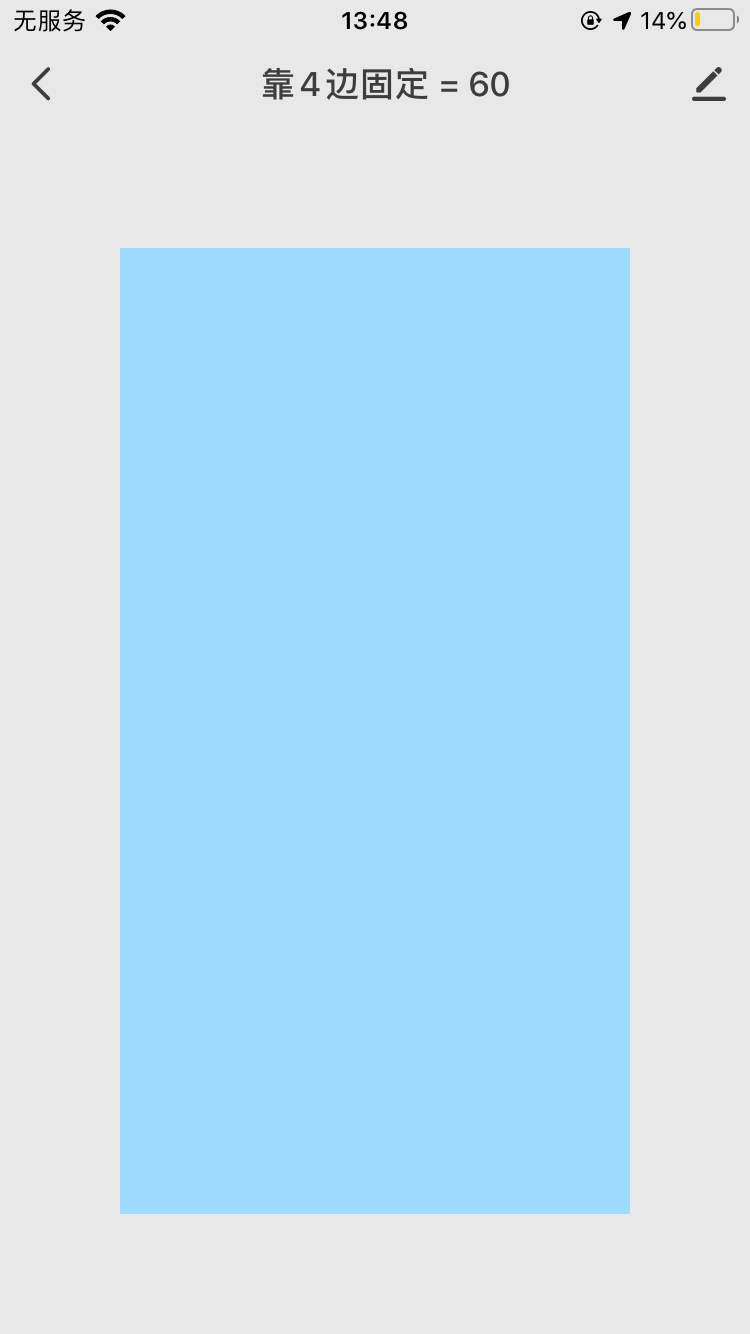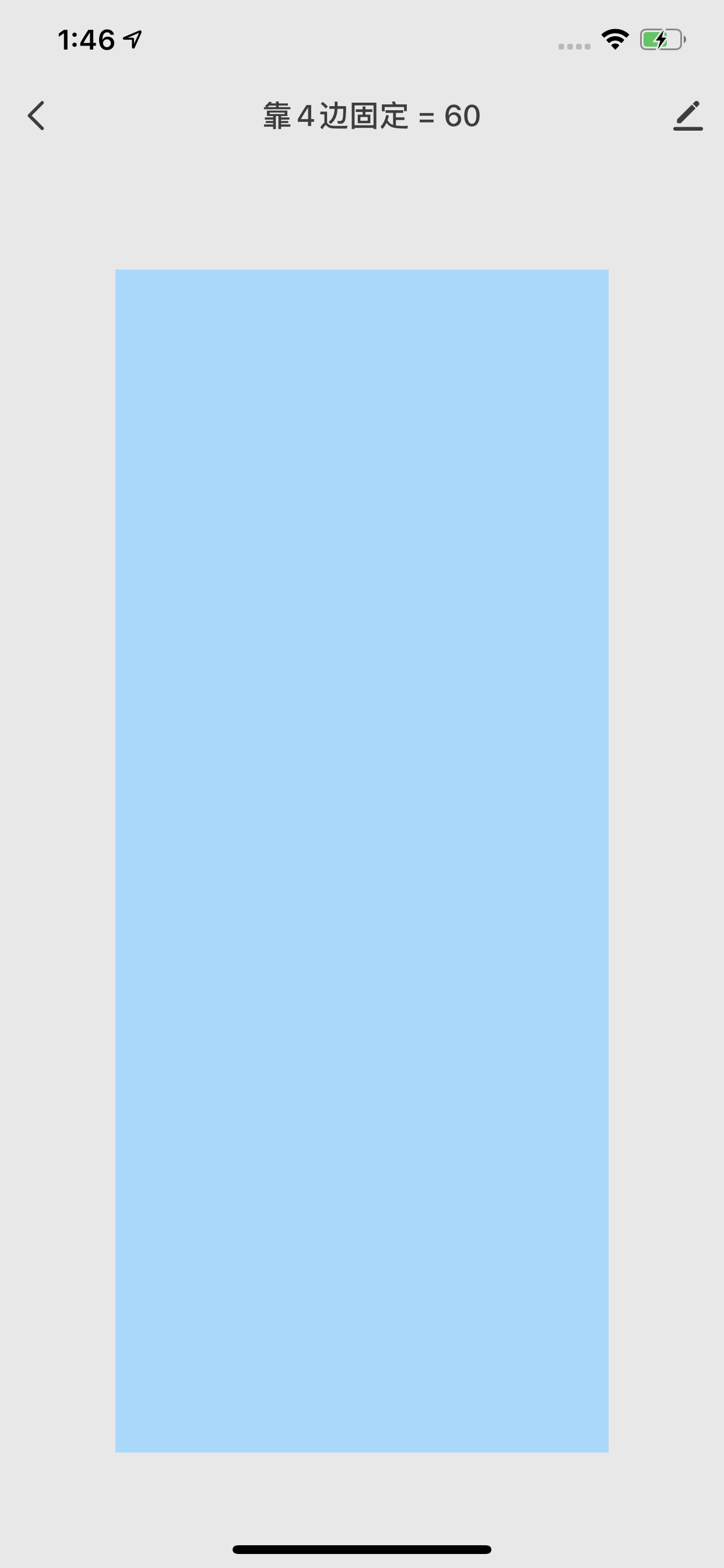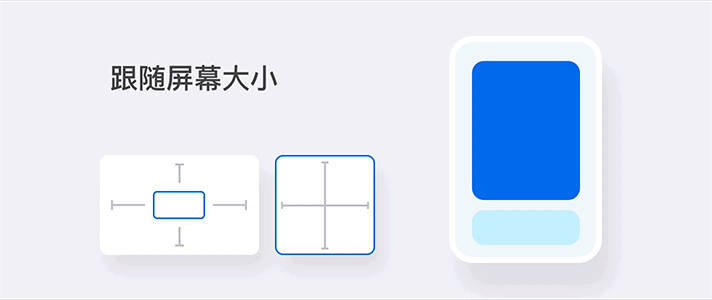# 响应式布局

## 响应式布局• 靠边固定：用来确定组件与画布（或矩形等区域）的固定比例的距离。当手机屏幕的长宽尺寸变化时，组件与画布的距离也会有一定程度的变化。

• 固定尺寸：组件本身的宽高等比例固定。当手机屏幕变大时，组件本身会相应的等比例变大。

• 配置项：

• 靠边固定：固定顶部，固定底部，固定左侧，固定右侧
• 固定尺寸：固定宽度，固定高度

### 应用场景

1. 拖一个布局组件”滚动“到画布。
2. ”滚动“ 组件配置项 ”布局“ 设置为 —— 固定左 = 固定右 = 固定顶 = 固定底 = 60 。
3. 此时在不同的手机屏幕上（ 短屏幕：iPhone 6s；长屏幕：iPhone XS Max ）的效果如下：### 配置说明

• 从水平方向看：固定左侧、固定右侧、固定宽度
• 从垂直方向看：固定顶部、固定底部、固定高度

• 水平：左+宽，右+宽，左+右
• 垂直： 顶+高，底+高，顶+底

## 预设布局的配置原理

- 固定左=固定右=固定顶=固定底 =0

- 固定左=固定右=固定顶=0；

- 固定左=固定右=固定底=0

- 固定左=固定右=固定顶=0；

- 固定左=固定右=固定底=0

- 固定左=固定右=固定顶=0；

- 固定左=固定右=固定底=0

- 固定左=固定右=固定顶=0；

- 固定左=固定右=固定底=0

- 固定左=固定右=固定顶=固定底 =0，可以拖曳布局组件进行自定义